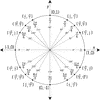# Unit Circle Labeled With Special Angles And ValuesIllustration of a unit circle (circle with a radius of 1) superimposed on the coordinate plane with the x- and y-axes indicated. The circle is marked and labeled in both radians and degrees at all quadrantal angles and angles that have reference angles of 30°, 45°, and 60°. At each angle, the coordinates are given. These coordinates can be used to find the six trigonometric values/ratios. The x-coordinate is the value of cosine at the given angle and the y-coordinate is the value of sine. From those ratios, the other 4 trigonometric values can be calculated.

Unit Circles

### Source

Florida Center for Instructional Technology Clipart ETC (Tampa: University of South Florida, 2007)

EPS (vector)

533.3 KiB

TIFF (full resolution)

3000×3000, 431.1 KiB

Large GIF

1024×1024, 56.9 KiB

Medium GIF

640×640, 33.0 KiB

Small GIF

320×320, 13.3 KiB